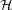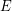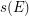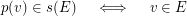# Straight line representation of planar linear hypergraphs (Solved)

 Importance: Medium ✭✭
 Author(s): de Fraysseix, Hubert Ossona de Mendez, Patrice
 Subject: Graph Theory » Topological Graph Theory » » Drawings
 Keywords: intersection graph planar hypergraph
Conjecture   Every planar linear hypergraphhas a straight line representation in the plane which maps each vertexto a pointand each edgeto a straight line segment, in such a way that:
\item for each vertexand each edge, we have:\item for each couple of distinct edges, we have